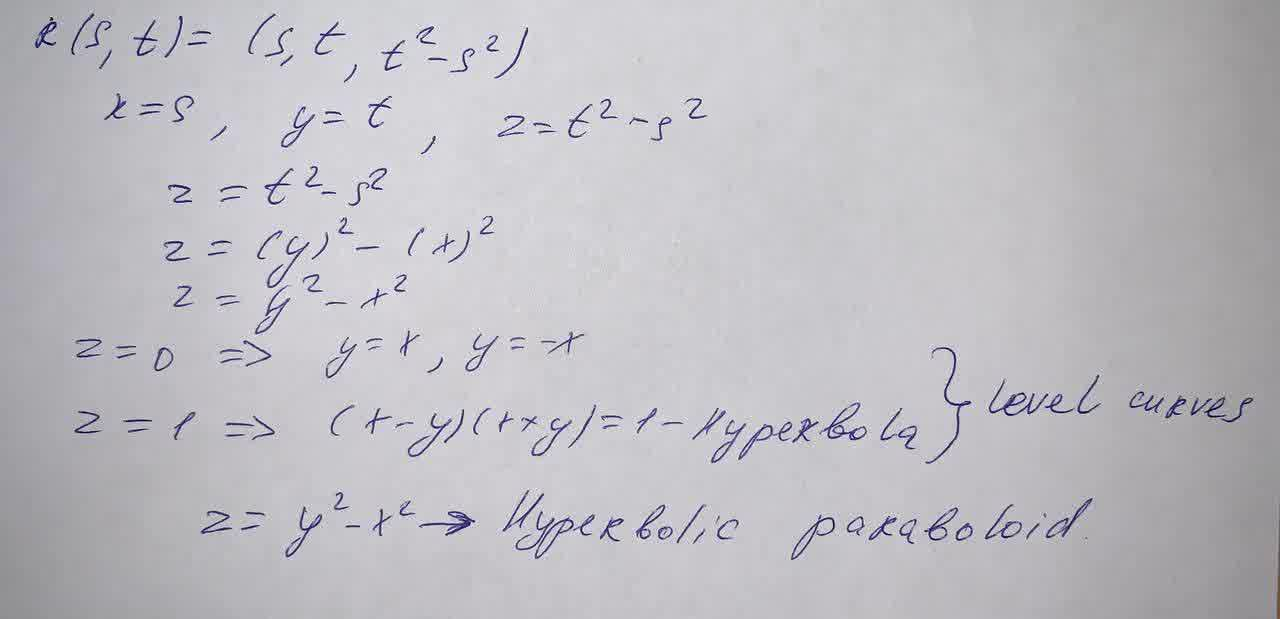Identify the surface with the given vector equation.r(s,t)=(s,t,t^2-s^2)eliptic cylindercircular paraboloidhyperbolic paraboloidplanecircular cylinderCarol Gates 2021-05-01 Answered

Identify the surface with the given vector equation. $$r(s,t)=$$$$(s,t,t^2-s^2)$$
eliptic cylinder
circular paraboloid
hyperbolic paraboloid
plane
circular cylinder

• Questions are typically answered in as fast as 30 minutes

Solve your problem for the price of one coffee

• Math expert for every subject
• Pay only if we can solve itMalena